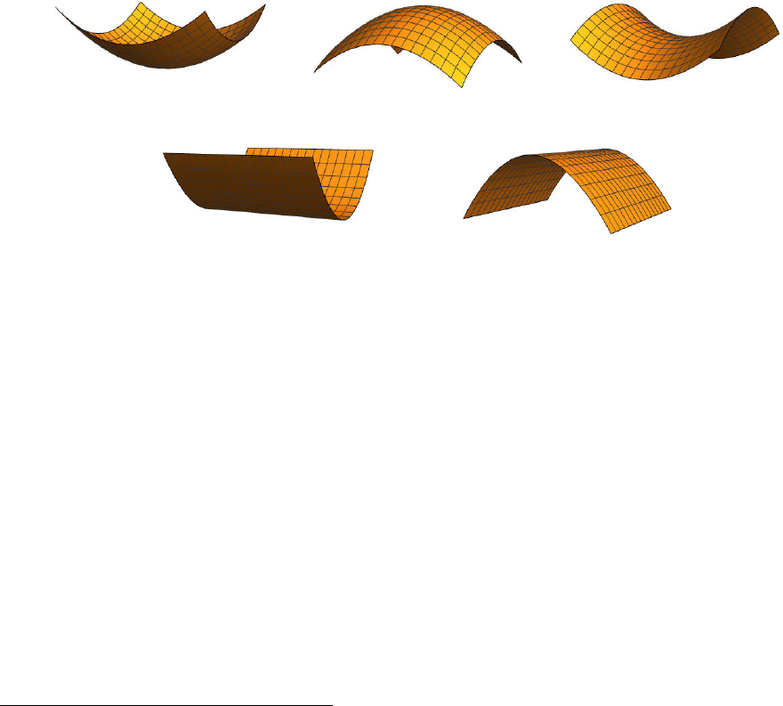Study Guides (380,000)
US (220,000)
UCLA (3,000)
MATH (300)
All (20)
Final

MATH 33AH Lecture Notes - Lecture 23: A Priori And A Posteriori, Diagonalizable Matrix, Orthogonal MatrixExam

Department
Mathematics
Course Code
MATH 33AH
Professor
All
Study Guide
Final

This preview shows page 1. to view the full 4 pages of the document.Math 33A Week 10
November 29 and December 1, 2016
Given a quadratic form q(~x), we often care about the range of values the form might take.
A priori, we know a few things about the values of q. We always have q(~
0) = 0, and the
range of qis unbounded, since
q(k~x) = k2~x
for any scalar kR. Questions whose answer we don’t know, however, include whether or
not qtakes on any negative values, or whether qhas a nontrivial kernel. Figure 1 shows
plots of various forms q:R2R.
(a) A positive-deﬁnite form. (b) A negative-deﬁnite form. (c) An indeﬁnite form.
(d) A positive semi-deﬁnite form. (e) A negative semi-deﬁnite form.
Figure 1: Plots of quadratic forms.
In these plots we see ﬁve distinct behaviors. The form in Figure 1a never takes on a
negative value, and the only vector in its kernel is the zero vector. That is, q(~x)>0 for
all nonzero vectors ~x. On the other hand, Figure 1b has the property that q(~x)<0 for all
nonzero vectors ~x. We say that these forms are positive-deﬁnite and negative-deﬁnite,
respectively. Figure 1c shows a form that takes on both positive and negative values, and
we say that such a form is indeﬁnite. The forms in Figures 1d and 1e are similar to those
in Figures 1a and 1b, respectively, in that their range is of the form [0,) or (−∞,0]. The
diﬀerence, though, is that the forms in Figures 1d and 1e both have nontrivial kernels. We
say that the form in Figure 1d is positive semi-deﬁnite, meaning that q(~x)0 for all ~x,
but that there is some nonzero vector ~x so that q(~x) = 0. Similarly, the form in Figure 1e
is called negative semi-deﬁnite. Our goal now is to classify quadratic forms according to
these ﬁve categories. In particular, we want to take a quadratic form q(~x) = ax2
1+bx1x2+cx2
2
and determine which of these ﬁve terms is in eﬀect1.
1Of course, there’s one form which doesn’t fall into any of these ﬁve categories: the form q(~x) = 0.
1